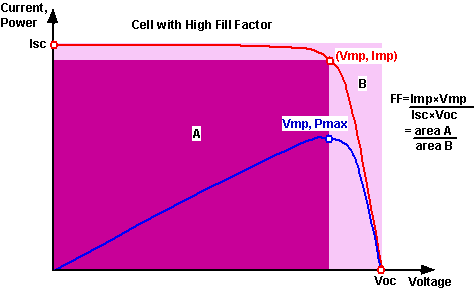# Fill Factor

The short-circuit current and the open-circuit voltage are the maximum current and voltage respectively from a solar cell. However, at both of these operating points, the power from the solar cell is zero. The "fill factor", more commonly known by its abbreviation "FF", is a parameter which, in conjunction with Voc and Isc, determines the maximum power from a solar cell. The FF is defined as the ratio of the maximum power from the solar cell to the product of Voc and Isc so that:

$$FF= \frac{P_{MP}}{V_{OC}\times I_{SC}}$$

## Fill Factor

$FF=\frac{{V}_{MP}{I}_{MP}}{{V}_{OC}{I}_{SC}}$

Graphically, the FF is a measure of the "squareness" of the solar cell and is also the area of the largest rectangle which will fit in the IV curve. The FF is illustrated below.Graph of cell output current (red line) and power (blue line) as a function of voltage. Also shown are the cell short-circuit current (Isc) and open-circuit voltage (VOC) points, as well as the maximum power point (Vmp, Imp). Click on the graph to see how the curve changes for a cell with low FF.

As FF is a measure of the "squareness" of the IV curve, a solar cell with a higher voltage has a larger possible FF since the "rounded" portion of the IV curve takes up less area. The maximum theoretical FF from a solar cell can be determined by differentiating the power from a solar cell with respect to voltage and finding where this is equal to zero. Hence:

$$\frac{d(I V)}{d V}=0$$

giving:

$$V_{MP} = V_{OC} - \frac{nkT}{q}ln(\frac{q V_{MP}}{nkT}+1)$$

It is an implicit equation, but it converges rapidly with iteration. By starting with VMP = 0.9 × VOC as the initial condition, there is < 1% error after one iteration and negligible (< 0.01%) after three iterations. An alternative is using Lambert functions (see below). Substituting the value of VMP back into the diode equation gives IMP and then FF. A more commonly used empirical expression for the FF is:1

## Fill Factor - Empirical

$FF=\frac{{v}_{oc}-\mathrm{ln}\left({v}_{oc}+0.72\right)}{{v}_{oc}+1}$

where voc is defined as a "normalized Voc":

## Voc normalized

${v}_{oc}=\frac{q}{nkT}{V}_{oc}$

Fill Factor Calculator 1

###### Results

Normalized VOC, voc (units):X
Fill Factor, FF:X

The above equations show that a higher voltage will have a higher possible FF. However, large variations in open-circuit voltage within a given material system are relatively uncommon. For example, at one sun, the difference between the maximum open-circuit voltage measured for a silicon laboratory device and a typical commercial solar cell is about 120 mV, giving maximum FF's respectively of 0.85 and 0.83. However, the variation in maximum FF can be significant for solar cells made from different materials. For example, a GaAs solar cell may have a FF approaching 0.89.

The above equation also demonstrates the importance of the ideality factor, also known as the "n-factor" of a solar cell. The ideality factor is a measure of the junction quality and the type of recombination in a solar cell. For the simple recombination mechanisms discussed in Types of Recombination, the n-factor has a value of 1. However, some recombination mechanisms, particularly if they are large, may introduce recombination mechanisms of 2. A high n-value not only degrades the FF, but since it will also usually signal high recombination, it gives low open-circuit voltages.

A key limitation in the equations described above is that they represent a maximum possible FF, although in practice the FF will be lower due to the presence of parasitic resistive losses, which are discussed in Effects of Parasitic Resistances. Therefore, the FF is most commonly determined from measurement of the IV curve and is defined as the maximum power divided by the product of Isc*Voc, i.e.:

## Fill Factor

$FF=\frac{{V}_{MP}{I}_{MP}}{{V}_{OC}{I}_{SC}}$

Fill Factor Calculator 2

###### Results

Resulting fill factor, FF: X

### Exact determination of VMP

The equation for a solar cell is:

$$I = I_L-I_0\left[\exp\left(\frac{V}{nV_t}\right)-1\right]$$

$$Power = V \times I$$ and in addition the -1 term has no effect at VMP

$$P = V I_L- V I_0\exp\left(\frac{V}{nV_t}\right)$$

VMP is when the derivative of the power with respect to V is zero:

$$0 = I_L- I_0\exp\left(\frac{V_{MP}}{nV_t}\right)\left(1+\frac{V_{MP}}{nV_t}\right)$$

V> > Vt and rearranging gives:

$$\frac{I_L}{I_0} =\exp\left(\frac{V_{MP}}{nV_t}\right)\left(\frac{V_{MP}}{nV_t}\right)$$

The Lambert W function provides the solution to a class of exponential functions.

$$Y = Xe^x \Leftrightarrow X = W (Y)$$

so we get:

$$\frac{V_{MP}}{nV_t} =W\left(\frac{I_L}{I_0}\right)$$

$$V_{MP} = nV_t W\left(\frac{I_L}{I_0}\right)$$

using the expression for VOC we can also write:

$$V_{MP} = nV_t W\left(\exp\left(\frac{V_{OC}}{nV_t}\right)\right)$$

Since the expression inside the W() is always real and positive we only ever need the principle branch of the Lambert W function, W0. The Lambert W function is a transcendental function much like logarithm function. While it is not available on most calculators, it is available on advanced mathematical packages such as Matlab or Python. With further analysis the Lambert W function can also be used for other solar cell terms and in the presence of parasitic resistances 2.